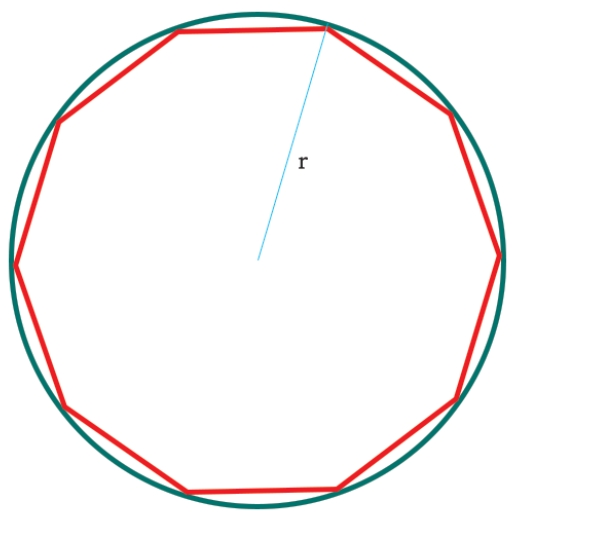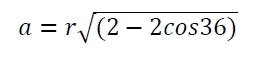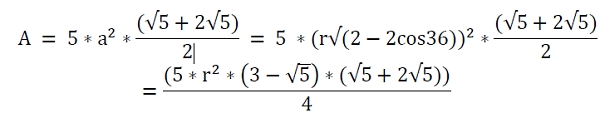# C Program for area of decagon inscribed within the circle?

CServer Side ProgrammingProgramming

Here we will see how to get the area of decagon which is present inside the circle. The radius is given. The side of the decagon is ‘a’.As we know that the side of the decagon is like below −So the area is −## Example

#include <iostream>
#include <cmath>
using namespace std;
float area(float r) {
if (r < 0) //if r is negative it is invalid
return -1;
float area = (5 * pow(r, 2) * (3 - sqrt(5)) * (sqrt(5) + (2 * sqrt(5)))) / 4;
return area;
}
int main() {
float r = 8;
cout << "Area : " << area(r);
}

## Output

Area : 409.969